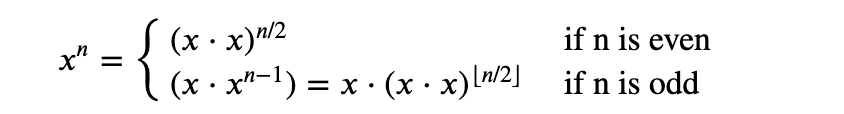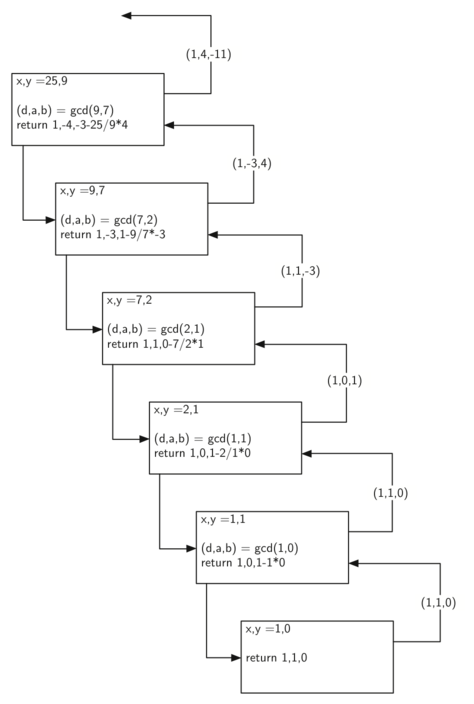# Luna Tech

Tutorials For Dummies.

# 0. 前言

cryptography（密码学）在互联网上使用的非常广泛。每次你登录到一个网站，都会用到 cryptography。

# 1. Modulo Operator

• a 除以 b 的余数在 0 到 (b-1) 之间
• 任何数除以 10 的余数都是 0-9 之间

def encrypt(m):
s = "abcdefghijklmnopqrstuvwxyz"
n = ""
for i in m:
j = (s.find(i) + 13) % 26
n = n + s[j]
return n


In addition, since there are 26 letters in the alphabet, this function is symmetric. The symmetry allows us to use the function to encrypt and decrypt the same message. If you pass the string "uryybjbeyg" to the encrypt function it returns "helloworld".

## Why 13?

13 是 26/2 的结果，也是它保证了上述 encrypt function 的对称性。

Asymmetry would require us to write a separate decrypt algorithm that subtracted the amount to rotate.

# m: message
# k: encryption rotate number
# (26-k): decrypt rotate number

def encrypt(m, k):
s = "abcdefghijklmnopqrstuvwxyz"
n = ""
for i in m:
j = (s.find(i) + k) % 26
n = n + s[j]
return n

def decrypt(m, k):
s = "abcdefghijklmnopqrstuvwxyz"
n = ""
for i in m:
j = (s.find(i) + 26 - k) % 26
n = n + s[j]
return n


# 2. Modular Arithmetic Theorems 同余定理

• 𝑎≡ 𝑏 (mod 𝑛)

1. If 𝑎≡𝑏 (mod 𝑛) then ∀𝑐, 𝑎+𝑐≡𝑏+𝑐 (mod 𝑛).
2. If 𝑎≡𝑏 (mod 𝑛) then ∀𝑐, 𝑎𝑐≡𝑏𝑐 (mod 𝑛).
3. If 𝑎≡𝑏 (mod 𝑛) then ∀𝑝, 𝑝>0, $𝑎^𝑝$≡$𝑏^𝑝$ (mod 𝑛).

∀ - for all

• 假如 a 和 b 是 n 的同余数，那么 a+c 和 b+c 也是 n 的同余数；
• 假如 a 和 b 是 n 的同余数，那么 a 乘以 c 和 b 乘以 c 也是 n 的同余数；
• 假如 a 和 b 是 n 的同余数，那么当 p > 0 的时候，a 的 p 次方和 b 的 p 次方也是 n 的同余数。

## Modular Exponentiation （模密）

• 假如 a 和 b 是 n 的同余数，那么当 p > 0 的时候，a 的 p 次方和 b 的 p 次方也是 n 的同余数。

### 步骤

• 先把 result 乘以 x；
• 再对 result 取余数；

The above approach makes the computation simpler because we are keeping the result smaller rather than following it out to its full precision. However, we can do even better using a recursive approach.

### 进阶版（递归）Remember that for a floating point number 𝑛 the floor operation, ⌊𝑛⌋, results in the largest integer smaller than 𝑛.

Python’s integer division operator returns the floor of the result of the division, so we do not need to do anything special in our code to achieve the results we want.

Python 的整数除法运算会返回结果的 floor result，所以我们直接用就行了。

The above equation gives us a very nice recursive definition for computing $𝑥^n$. All we need now is a base case.

Also note that since we are computing modulo p we still apply the modulo operator at each step of the calculation. The solution in keeps the result size small and uses many fewer multiplications than a purely iterative approach.

def modexp(x, n, p):
if n == 0:
return 1
t = (x * x) % p
tmp = modexp(t, n // 2, p)
if n % 2 != 0:
tmp = (tmp * x) % p
return tmp


# 3. 最大公约数 The Greatest Common Divisor

## Multiplicative Inverses 模反元素/模倒数/模逆元

A multiplicative inverse of a positive integer 𝑥 modulo 𝑚 is any number 𝑎 such that 𝑎𝑥≡1(mod 𝑚).

3 * 5 = 15，15 % 7 = 1

5 就是 3 除以 7 取余（3）的一个模反元素。

for i in range(1, 40):
if (3 * i) % 7 == 1:
print i # 5,12,19,26,33


## 所有数字都有模反元素吗？

Do all pairs of numbers 𝑥 and 𝑚, have a multiplicative inverse?

(4*i)/8 的结果只能是 0 或者 4，不可能是 1。

## 那么，到底哪些数字有模反元素呢？

The answer is that a number 𝑥 has a multiplicative inverse, modulo 𝑚, if and only if 𝑚 and 𝑥 are relatively prime.

## 那么，哪些数字互为质数呢？

Two numbers are relatively prime if 𝑔𝑐𝑑(𝑚,𝑥)=1

# 4. 我们如何计算两个数字的最大公约数呢？

## 欧几里得算法

Given two numbers 𝑎 and 𝑏 we can find the GCD by repeatedly subtracting 𝑏 from 𝑎 until 𝑎<𝑏. When 𝑎<𝑏, we switch roles for 𝑎 and 𝑏. At some point 𝑎−𝑏 becomes 0, so we swap 𝑎 and 𝑏 one more time. At that point we have 𝑔𝑐𝑑(𝑎,b)=𝑎. This algorithm was first described more than 2,000 years ago and is called Euclid’s Algorithm.

• 计算 a - b 的值，直到 a 小于 b；
• 当 a 小于 b 的时候，a 和 b 互换；
• 当 a - b 等于 0 的时候，互换 a 和 b，a 和 b 的最大公约数就是 a。

1. a = 7, b = 3
2. a - b = 7 - 3 = 4，a = a - b = 4
3. 因为 4 > b，所以计算 4 - b = 4 - 3 = 1
4. 因为 1 < b，所以互换 a 和 b，a = 3, b = 1
5. 计算 a - b = 2，a = 2，b = 1
6. 因为 2 > 1，所以计算 2 - 1 = 1,
7. 此时 a = b = 1，a - b = 0
8. a 和 b 互换（这里换不换都一样，都是1），所以 3 和 7 的最大公约数为 1，两数互为质数
def gcd(a, b):
if b == 0:
return a
elif a < b:
return gcd(b, a)
return gcd(a - b, b)


## 辗转相除法

def gcd(a, b):
if b == 0:
return a
return gcd(b, a % b)


# 5. 计算模反元素

m = 3, x = 7, a = -2, b = 1

𝑏𝑥 - 1 = 1*7 - 1 = 6

(bx -1) mod 𝑚 = 6 mod 3 = 0

b 是 x 对模数 m 的模反元素。

# 6. 欧几里得算法拓展

We have reduced the problem of computing inverses to the problem of finding integers 𝑎 and 𝑏 that satisfy the equation 𝑑=𝑔𝑐𝑑(𝑥,𝑦)=𝑎𝑥+𝑏𝑦.

We will take two numbers 𝑥>=𝑦 and return a tuple (𝑑,𝑎,𝑏) such that 𝑑=𝑔𝑐𝑑(𝑥,𝑦) and 𝑑=𝑎𝑥+𝑏𝑦.

d 是 x 和 y 的最大公约数，ax + by 等于 d。

def ext_gcd(x, y):
if y == 0:
return (x, 1, 0)
else:
(d, a, b) = ext_gcd(y, x % y)
return (d, b, a - (x // y) * b) # 后面证明


## 图解拓展算法## 说明

• x = 25，y = 9

• base case 是 y = 0，我们返回 d = x（和原始版的欧几里得算法一样）；

我们同时还返回 a = 1，b = 0，这三个值是满足 ax + by = d 这个等式的。

• 假如 y > 0，我们就递归计算 d, a, b 三个值，让它们满足 𝑑=𝑔𝑐𝑑(𝑦,𝑥mod𝑦) and 𝑑=𝑎𝑦+𝑏(𝑥mod𝑦)

和原始算法一样，𝑑=𝑔𝑐𝑑(𝑥, 𝑦) ；

a 和 b 都必须是整数；

整理一下算式：

𝑑 = 𝑎𝑦+𝑏(𝑥mod𝑦)

= 𝑎𝑦+𝑏(𝑥−⌊𝑥/𝑦⌋𝑦)

= 𝑏𝑥+(𝑎−⌊𝑥/𝑦⌋𝑏)𝑦

为什么 𝑏(𝑥mod𝑦) 可以被替换为 𝑏(𝑥−⌊𝑥/𝑦⌋𝑦)？

因为这就是我们计算 (𝑥mod𝑦) 的方式。

整理完我们可以发现，最终输出的结果 A = b，B = (𝑎−⌊𝑥/𝑦⌋𝑏)。

也就是我们代码最后一行 return 的逻辑：

return (d, b, a - (x // y) * b)

• 我们每一步返回的值都满足 ax + by = d （可以参考上图）